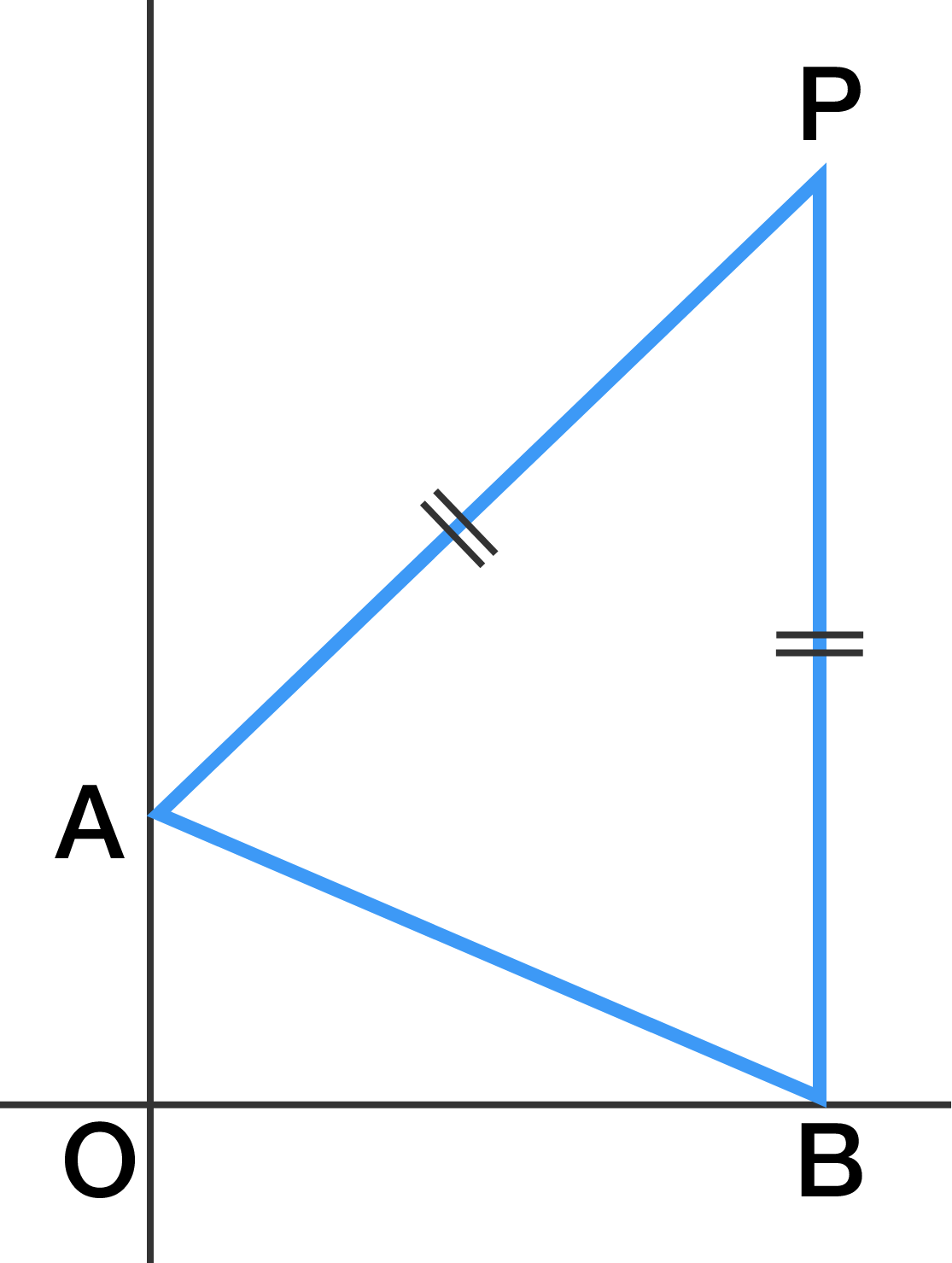# The Tale of Point P

Geometry Level 1The $\triangle AOB$ consists of point $A = (0 , 1)$, point $O = (0 , 0)$, and point $B$ lying somewhere on the $x$-axis.

Let $P$ be the point in the first quadrant such that $AP = PB$ and $AO \parallel PB$, as shown above.

If the length of $OP$ is $\sqrt{194}$, what is the area of the quadrilateral $AOBP?$

×